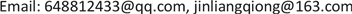SAStatistics and Application2325-2251Scientific Research Publishing10.12677/SA.2019.82040SA-29913SA20190200000_47627818.pdf数学与物理 基于分位数回归模型的VaR研究——以贵州百灵股票为例 VaR Research Based on Quantile Regression Model—Taking Guizhou Bailing Stock as an Example 仕彤21良琼21贵州民族大学，数据科学与信息工程学院，贵州 贵阳null290320190802364369© Copyright 2014 by authors and Scientific Research Publishing Inc. 2014This work is licensed under the Creative Commons Attribution International License (CC BY). http://creativecommons.org/licenses/by/4.0/

VaR风险测度，分位数回归方法，GARCH模型, Value-at-Risk Quantile Regression Method Garch Model

——以贵州百灵股票为例Copyright © 2019 by authors and Hans Publishers Inc.1. 引言

2. 基于分位数回归模型的VaR风险测度

F − 1 ( τ ) = inf { y | F ( y ≥ τ ) } (1)

X 是 p 维回归变量，当 X = x 时，定义 Y 的条件分布为：

F Y − 1 ( τ | x ) = x T β ( τ ) (2)

min β ∑ t = 1 n ρ τ ( y t − x t T β ) (3)

ρ ( τ ) ( u ) = { τ u , u ≥ 0 ( 1 − τ ) u , u < 0 (4)

Q y t ( τ | x i ) = inf { y ∈ R : F ( y | x ) ≥ τ } ≡ F − 1 ( τ | x ) (5)

Q y t ( τ | x i ) = x T β ( τ ) (6)

V a R τ = Q y t ( τ | x i ) (7)

Q y t ( τ | y t − 1 , y t − 2 , ⋯ , y t − m ) = α 0 + α 1 y t − 1 + y t − 2 + ⋯ + α m y t − m (8)

3. 基于GARCH模型的VaR风险测度

{ y t = X ′ t + ε t , ε t = σ t η t σ t 2 = ω + ∑ i = 1 q α i ε t − i 2 + ∑ i = 1 p β i σ t − i 2 (9)

σ t 2 = ω + α 1 ε t − 1 2 + β 1 σ t − 1 2 (10)

k = E ( ε t 2 ) [ E ( ε t 2 ) ] 2 = 3 [ 1 − ( α 1 + β 1 ) 2 ] 1 − 2 α 1 2 − ( α 1 + β 1 ) 2 > 3 (11)

y t = ϕ 0 + ∑ i = 1 m ϕ i y t − i + a t − ∑ j = 1 n θ j a t − j , a t = σ t ε t (12)

σ t 2 = ω + ∑ i = 1 p α i a t − i 2 + ∑ j = 1 q β j σ t − j 2 (13)

y ^ t ( 1 ) = ϕ 0 + ∑ i = 1 m ϕ i y t + 1 − i − ∑ j = 1 n θ j a t + 1 − j (14)

σ ^ t 2 ( 1 ) = ω + ∑ i = 1 p α i a t + 1 − i 2 − ∑ j = 1 q β j σ t + 1 − j 2 (15)

VaR 1 − τ = y ^ t ( 1 ) + t 1 − τ ( s ) s / ( s − 2 ) σ ^ ( 1 ) (16)

4. 实证研究

4.1. 分位数回归的VaR模型

Q ( τ | y t − i ) = 3.8070756 + 0.25801940 y t − 1 + 0.32799306 y t − 2 + 0.0950663 y t − 3 (17)

4.2. GARCH的VaR模型

The result of the basic characteristic of logarithmic rate of retur

r t0.15011−11.574929.285741541.827 (0.0000)3.02715 (0.0000)

{ r t = σ t ε t , ε t ~ ( 4.8670 ) σ t 2 = 0.12977 + 0.07301 ε t − 1 2 + 0.9154 σ t − 1 2 (18)

5. 总结

VaR已然成为当今国际上最为主流的一种有效的金融风险度量的工具，被金融各界人士广泛运用。文章基于分位数回归模型，对贵州百灵股票的VaR进行测度研究，通过Kupiec似然比检验将模型一与GARCH模型作了对比，得出GARCH模型失败率要高于模型一，故分位数回归模型在对VaR的风险测度研究上要比GARCH模型略稳定。当然由于金融数据本身的特点过于复杂，也意味着金融风险测度不会是一个简单的问题。虽然它的计算方法随着研究人员们的不断努力在不停地改善着，但时至今日，大部分的方法、模型也都是为VaR的测度研究提供参考。